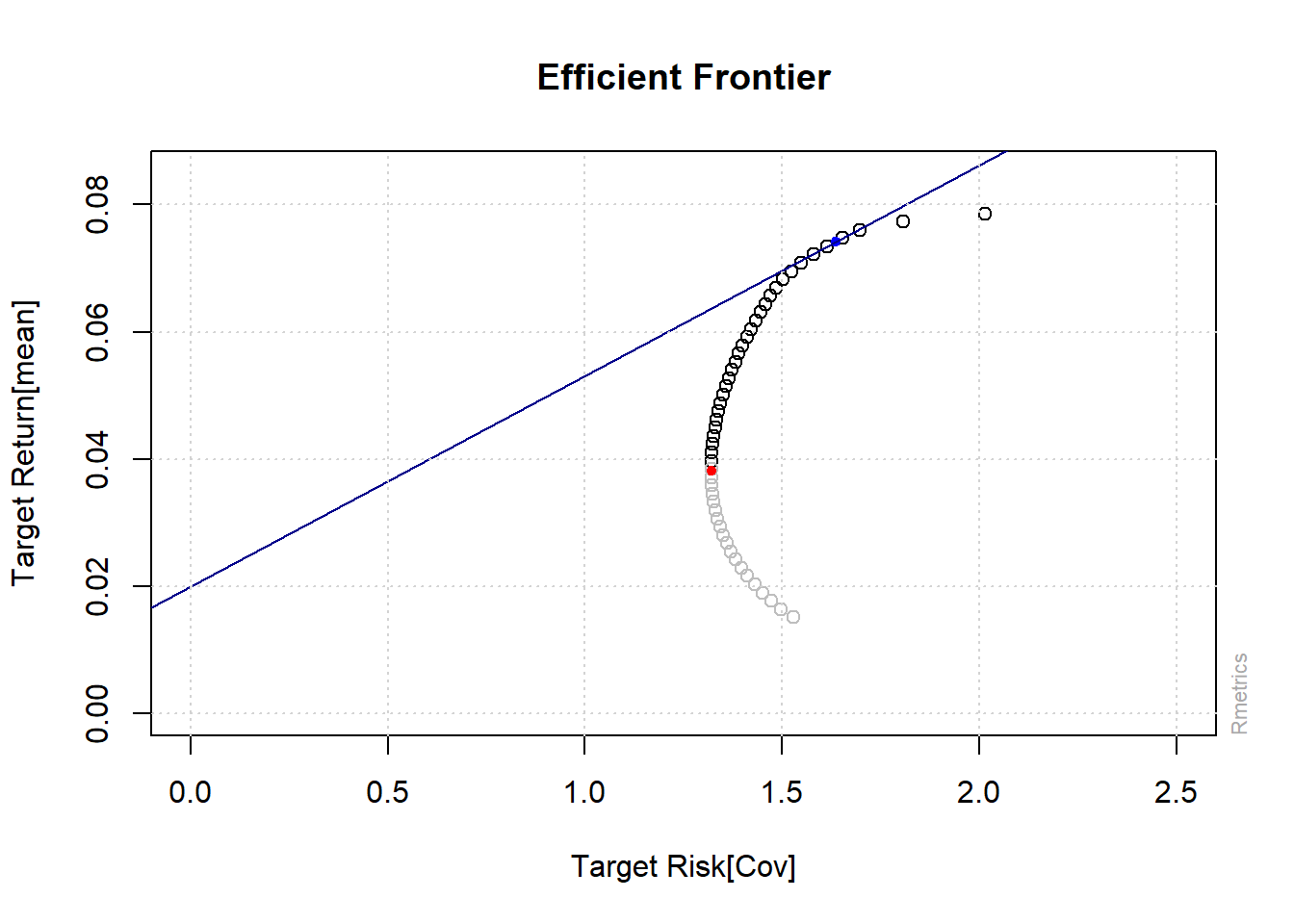## 12.7 Evaluating Portfoios: Risk Adjusted Performance

### 12.7.1 Sharpe Ratio

• The Sharpe Ratio compares the excess return of a portfolio relative to the risk-free rate with the portfolio’s standard deviation.
$\begin{equation} Sharpe_{p}=\frac{E(r_{p})-r_{f}}{\sigma_{p}} \tag{12.22} \end{equation}$
• The portfolio with highest Sharpe Ratio is known as the Tangency Portfolio

### 12.7.2 Roy’s Safety Ratio

• Roy’s Safety First (SF) Ratio makes a slight modification to the Sharpe Ratio.

• Specifically, instead of using the risk-free rate, Roy’s SF Ratio instead uses a target or minimum acceptable return to calculate the excess return

$\begin{equation} RoysSF_{p}=\frac{E(r_{p})-MAR}{\sigma_{p}} \tag{12.23} \end{equation}$

### 12.7.3 Treynor’s Ratio

• The Treynor Ratio modifies the denominator in the Sharpe Ratio to use beta instead of standard deviation in the denominator. That is
$\begin{equation} Treynor_{p}=\frac{E(r_{p})-r_{f}}{\beta_{p}} \tag{12.24} \end{equation}$

### 12.7.4 Sharpe Ratio (Portfolio) in R

• Assume an rf=0.02
• The code uses the fPortfolio package
• The ratio can also be easily calculated using base R
# create specification
pspec3 = portfolioSpec()
setRiskFreeRate(pspec3) = 0.02
setNFrontierPoints(pspec3) = 50
# create efficient frontier
eff_front4 = portfolioFrontier(data_p2, spec = pspec3, constraints = "LongOnly")

# find the tangency port
tgport1 = tangencyPortfolio(data = data_p2, spec = pspec3, constraints = "LongOnly")
# create frontier plot

frontierPlot(eff_front4, pch = 1, auto = FALSE, xlim = c(0, 2.5), ylim = c(0,
0.085))  #custom x and y limits
minvariancePoints(object = eff_front4, auto = FALSE, col = "red", pch = 20)  #add min variance port
tangencyPoints(object = eff_front4, col = "blue", pch = 20)  #add tangency port
tangencyLines(object = tgport1, col = "darkblue")  #add tangency portfolio line
grid()Figure 12.12: Tangency Portfolio (Sharpe Portfolio)

print(tgport1)

Title:
MV Tangency Portfolio
Estimator:         covEstimator
Optimize:          minRisk
Constraints:       LongOnly

Portfolio Weights:
BHP    ANZ    WOW    TLS    CSL
0.3996 0.0000 0.0758 0.0000 0.5247

Covariance Risk Budgets:
BHP    ANZ    WOW    TLS    CSL
0.3853 0.0000 0.0464 0.0000 0.5683

Target Returns and Risks:
mean    Cov   CVaR    VaR
0.0741 1.6352 4.0740 2.4228

Description:
Mon Oct 25 17:30:41 2021 by user: RMachine 
# weights plot weightsPie(tgport1,box=FALSE)
# weightedReturnsPie(tgport1,box=FALSE)